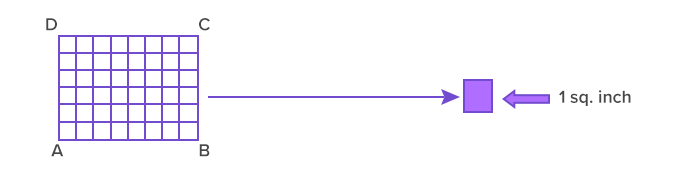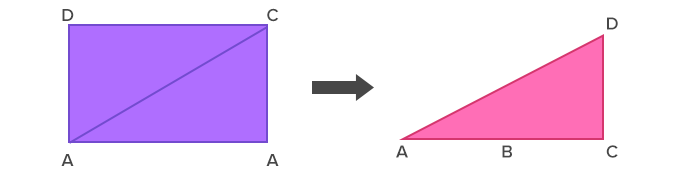Area Of Rectangle Formula - Definition with Examples

The Complete K-5 Math Learning Program Built for Your Child

• 30 Million Kids

Loved by kids and parent worldwide

• 50,000 Schools

Trusted by teachers across schools

• Comprehensive Curriculum

Aligned to Common Core

Area of Rectangle Formula

Keywords: rectangle, area, square inches, inches, perimeter, formula, length, square, multiply, polygon, find, area formula

A rectangle is a 2-dimensional shape/polygon with four sides, four vertices, and four right angles. The two opposite sides in the rectangle are equal and parallel to each other. The area of a rectangle is the space covered by the shape. Alternatively, the space within the perimeter of the rectangle is the area of a rectangle.

Some examples of rectangular figures are agricultural fields, parks, tiles, daily life objects such as pans, glass, table, serving tray, etc.The painting canvas and walkway with rectangular tiles (id= 1391133983, 443476687)

The upcoming section discusses the methods to understand why the area of a rectangle is the product of its two sides as well as the units of measurement.

Calculating the area of a rectangle

To derive the area of a rectangle, we use the unit squares. Divide the rectangle ABCD into unit squares, as shown. The area of a rectangle ABCD is the total number of unit squares contained within it.Rectangle ABCD in unit squares with each of 1 sq. inch

Thus, the total area of the rectangle ABCD is 48 sq. inch.

Also, using this approach, we find that the area of a rectangle is always the product of its two sides. Here, the length of AB is 8 inches and the length of BC is 6 inches. The area of ABCD is the product of 6 and 8, which is equal to 48.

The unit of measurement will be “square inches” as the lengths are multiplied together so are the units.

Alternatively, the formula to calculate the area of a rectangle is derived by dividing the shape into two equal size right triangles. For example, in the given rectangle ABCD, a diagonal from the vertex A is drawn to C.

The diagonal AC divides the rectangle into two equal right angle triangles.Thus, the area of ABCD will be:

⇒ Area (ABCD) = Area (ABC) + Area (ADC)

⇒ Area (ABCD) = 2 × Area (ABC)

⇒ Area (ABC) = 12 × base × height

⇒ Area (ABCD) = 2 × (1× b × h)

⇒ Area (ABCD) = b × h

Application

The early transcripts of Babylonian culture signify the use of geometric shapes with lengths, angles, and areas for construction and astronomy. The knowledge of stone cutting in basic shapes such as triangles, squares, and rectangles along with principles pertaining area and perimeter helped Egyptians to build giant structures like pyramids. In modern mathematics, these concepts are useful in map designing, land surveying, object modeling, and others.

 Fun Facts Both the diagonals of a rectangle are of equal length. A circle can contain a rectangle with all its vertex touching the circumference; it is called a cyclic rectangle.

Won Numerous Awards & Honors